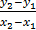### Sample Problem

Find the rate of change in the table below:

 4 6 8 9 18 27

#### Solution

Rate of change is the same as slope, so we will select 2 points in the table and calculate the slope between them

m =m =m =m = 4.5